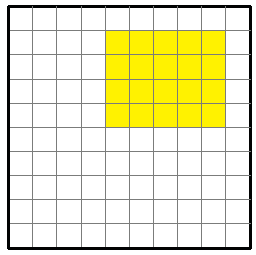# Finding the perimeter or area of a rectangle in the coordinate plane

#### Complete Python Prime Pack

9 Courses     2 eBooks

#### Artificial Intelligence & Machine Learning Prime Pack

6 Courses     1 eBooks

#### Java Prime Pack

9 Courses     2 eBooks

In this lesson, we are given rectangles in the coordinate plane. We are required to find the perimeters and the areas of these rectangles. We read the coordinates of the vertices of these rectangles and can count the lengths of the sides of the rectangles from the grid.

Once the lengths and widths are knows we can find the perimeters and areas of these rectangles.

Alternatively, we can count the number of unit squares on the grid to find the areas and along the boundary to find the perimeters of the rectangles.

Find the area and perimeter of the following rectangle.### Solution

Step 1:

Area of a rectangle = l × w; l = length = 5; w = width = 4

Step 2:

Perimeter of the rectangle = 5 + 4 + 5 + 4 = 18 units

Area of the rectangle = 5 × 4 = 20 square units.

Find the area and perimeter of the following rectangle.### Solution

Step 1:

Area of a rectangle = l × w; l = length = 7; w = width = 4

Step 2:

Perimeter of the rectangle = 7 + 4 + 7 + 4 = 22 units

Area of the rectangle = 7 × 4 = 28 square units.